Courses

# Test: Simple Stress & Strain Level - 3

## 20 Questions MCQ Test Engineering Mechanics | Test: Simple Stress & Strain Level - 3

Description
This mock test of Test: Simple Stress & Strain Level - 3 for Mechanical Engineering helps you for every Mechanical Engineering entrance exam. This contains 20 Multiple Choice Questions for Mechanical Engineering Test: Simple Stress & Strain Level - 3 (mcq) to study with solutions a complete question bank. The solved questions answers in this Test: Simple Stress & Strain Level - 3 quiz give you a good mix of easy questions and tough questions. Mechanical Engineering students definitely take this Test: Simple Stress & Strain Level - 3 exercise for a better result in the exam. You can find other Test: Simple Stress & Strain Level - 3 extra questions, long questions & short questions for Mechanical Engineering on EduRev as well by searching above.
QUESTION: 1

### Consider the following statements Modulus of rigidity and bulk modulus of a material are found to be 60 GPa and 140 GPa respectively. Then1. elasticity modulus is nearly 200 GPa2. Poisson’s ratio is nearly 0.33. elasticity modulus is nearly 158 GPa4. Poisson’s ratio is nearly 0.25Which of these statements are correct?

Solution: G = 60 GPa

K = 140 GPa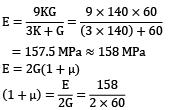μ = 0.31 ≈ 0.3 Statement 2 and 3 are correct.

QUESTION: 2

### In the arrangement as shown in the figure, the stepped steel bar ABC is loaded by a load P. The material has Young’s modulus E = 200 GPa and the two portions AB and BC have area of cross section 1cm2 and 2cm2 respectively. The magnitude of load P required to fill up the gap of 0.75mm is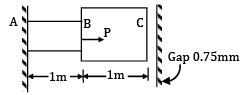Solution: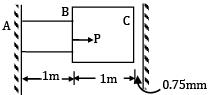E = 200 GPa = 200 x 103 MPa

AAB = 1 cm2 = 100 mm2

ABC = 2 cm2 = 200 mm2

Gap(δ) = 0.75 mm

There will be no deformation in BC so,

δAB = δ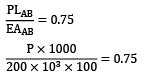P = 75 x 200 = 15 x 103N = 15 kN

QUESTION: 3

### An elastic material of Young’s modulus E and Poisson’s ratio v is subjected to a compressive stress of σ1 in the longitudinal direction.Suitable lateral compressive stress σ2 is also applied along the other two lateral directions to limit the net strain in each of the lateral directions to half of the magnitude that would be under σ1 acting alone. The magnitude of σ2 is

Solution: σ1 is acting along longitudinal direction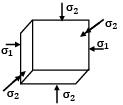Strain in lateral direction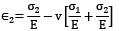Strain in lateral direction when σ1 acts alone.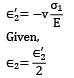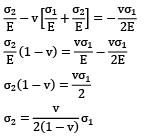QUESTION: 4

A spherical ball of steel of diameter 200 mm goes down to a depth of 1 km in sea water. If specific weight of sea water in 10.2 kN/m3, (and bulk modulus of steel is 170 kN/mm2), the change in volume of the spherical ball is ___________mm3

Solution: Depth, h = 1000 m

Weight density, w = 10.2 x 1000 N/m3

Pressure, p = wh = 1000 x 10.2 x 1000 N/m2

= 10.2 x 106 N/m2 Bulk modulus,

K = 170 x 103 N/mm2

= 170 x 109 N/m2

Volumetric strain,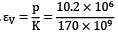=0.06 x 10-3

Original volume, V =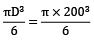=4.188 x 106mm3

Change volume,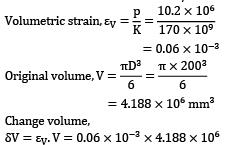= 0.25 x 103 mm3

= 250 mm3

Question_Type: 5

QUESTION: 5

A bar of length L tapers uniformly from diameter 1.1 D at one end to 0.9 D at the other end. The elongation due to axial pull is computed using mean diameter D. What is the approximate error in computed elongation?

Solution: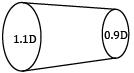Actual elongation,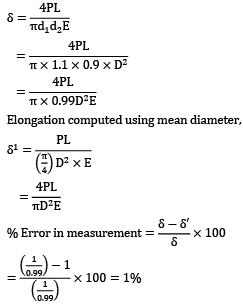Elongation computed using mean diameter,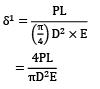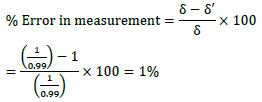QUESTION: 6

A cube having each side of length a, is constrained in all directions and is heated uniformly so that the temperature is raised by T℃ . If α is the thermal coefficient of expansion of the cube material and E the modulus of elasticity, the stress developed in the cube is

Solution: Since the cube is restrained in all directions,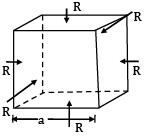⇒ εv = εx + εy + εz ⋯ ①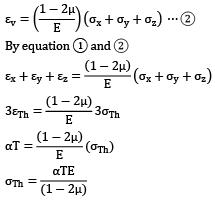QUESTION: 7

Consider the following statements: The thermal stress is induced in a component in general, when

1. a temperature gradient exists in the component

2. the component is free from any restraint

3. it is restrained to expand or contract freely

Which of the above statements are correct?

Solution:

1. If a temperature gradient exists in the component, then there will be thermal stresses generated if the component is restrained to expand or contract freely.

2. If the component is free from restraints, no stresses will be generated. However, strain will be there.

3. Thermal stresses will be induced when it is restrained to expand or contract freely.

Ex.: fixed beam with a rise in temperature.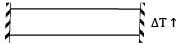QUESTION: 8

A straight bar is fixed at edges A and B. Its elastic modulus is E and cross-section is A. There is a load P = 120 N acting at C. Determine the reactions at the ends.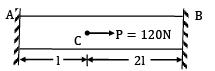Solution: The FBD can be shown as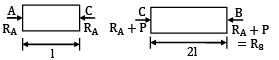δAC + δCB = 0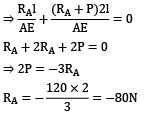(Opposite to the direction assumed)

⇒ RB = −80 + 120 = 40N

QUESTION: 9

A rigid beam of negligible weight is supported in a horizontal position by two rods of steel and aluminum, 2m and 1m long having values of cross-sectional areas 1 cm2 and 2 cm2 and E of 200 GPa and 100 GPa respectively. A load P is applied as shown in the figure.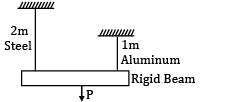If the rigid beam is to remain horizontal then

Solution: If the beam is to remain horizontal ⇒ δAB = δCD Let force on rod AB be P′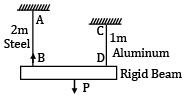⇒ Force on rod CD = P − P′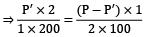⇒ 2P′ = P − P′

⇒ 3P′ = P

⇒ P′ = P/3(force on steel rod) Force on aluminum rod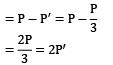⇒ Force on aluminum rod

= 2 x force on steel rod.

QUESTION: 10

Two tapering bars of the same material are subjected to a tensile load P. The lengths of both the bars are the same. The larger diameter of each of the bars is D. The diameter of the bar A at its smaller end is D/2 and that of the bar b is D/3. What is the ratio of elongation of the bar A to that of the bar B?

Solution: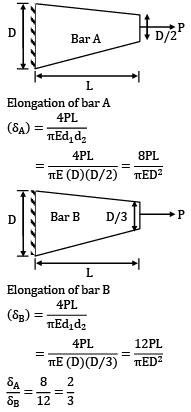Elongation of bar A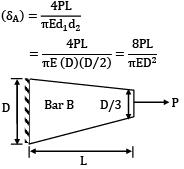Elongation of bar BQUESTION: 11

For a composite bar consisting of a bar enclosed inside a tube of another material and when compressed under a load W as a whole through rigid plates at the end of the bar. The equation of compatibility is given by (Suffixes 1 and 2 refer to bar and tube respectively)

Solution: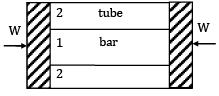Compatibility condition is

Elongation in bar = Elongation in tube

δ1 = δ2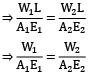QUESTION: 12

A tensile specimen with 12 mm initial diameter and 50 mm initial length is subjected to a load of 90 kN. After some time the diameter is 10 mm. Assuming it as incompressible material, Calculate the true strain along the length.

Solution: Given,

d1 = 12 mm

l1 = 50 mm p = 90 kN

d2 = 10 mm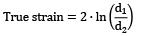= 0.36

Question_Type: 5

QUESTION: 13

If the rigid rod fitted snugly between the supports as shown in the figure below, is heated, the stress induced in it due to 20℃ rise in temperature will be (α = 12.5 x 10-6/℃ E = 200GPa)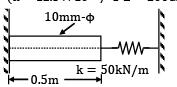Solution: Expansion of rod due to temperature rise δ = α∆tL

= 12.5 x 10−6 x 20 x 0.5

= 1.25 x 10−4m

Force induced by the spring due to compression

F = kδ = 50 x 103 x 1.25 x 10−4 = 6.25 N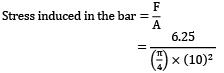This stress is compressive for the bar,

∴ Stress = −0.07945 MPa

QUESTION: 14

An aluminum bar of 8 m length and a steel bar of 5 mm longer in length are kept at 30℃.If the ambient temperature is raised gradually, at what temperature the aluminum bar will elongate 5 mm longer than the steel bar? (The linear expansion coefficients for steel and aluminum are 12 x 10−6/℃ and 23 x 10−6/℃ respectively)

Solution: L = 8 m Initially,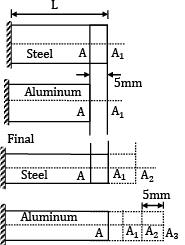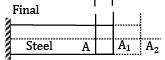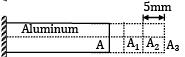αs = 12 x 10−6/℃

αa = 23 x 10−6/℃

t1 = 30℃

Total elongation of aluminum-total elongation of steel

= (AA3) − (A1A2)

= (AA1) + A1A2 + A2A3) − (A1A2)

= AA1 + A2A3 = 10mm

L αal∆t − Lαs∆t = 10

8 x 103 x ∆t[23 x 10−6 − 12 x 10−6] = 10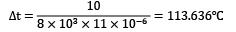t2 − 30° = 113.636℃

t2 = 143.636℃

QUESTION: 15

A 16 mm diameter bar elongates by 0.04% under a tensile force of 16kN. The average decrease in diameter is found to be 0.01%. Then

1. E = 210 GPa and G = 77 GPa

2. E = 199 GPa and V = 0.25

3. E = 199 GPa and V = 0.30

4. E = 199 GPa and G = 80 GPa

Which of these values are correct?

Diameter (d) = 16mm

% elongation = 0.04%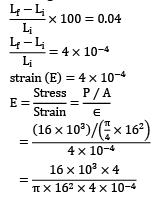= 198.94 x 103MPa

= 199 GPa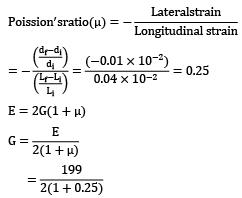= 79.6

= 80 GPa

Statement 2 and 4 are correct.

QUESTION: 16

The figure shows a steel piece of diameter 20 mm at A and C, and 10 mm at B. the lengths of three sections A, B and C are each equal to 20 mm. The piece is held between two rigid surface x and Y. The coefficient of linear expansion α = 1.2 x 10−5 /℃ and Young’s Modulus E = 2 x 105 MPa for steel,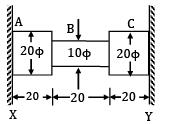When the temperature of this piece increase by 50℃, the stresses in sections A and B are

Solution: α = 1.2 x 10−5/℃

E = 2 x 105 MPa

∆T = 50℃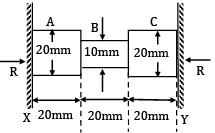Since supports are rigid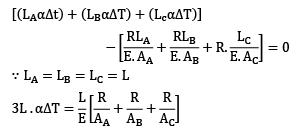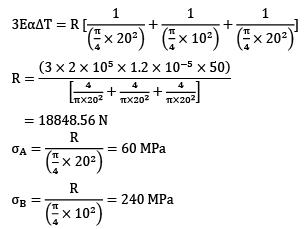QUESTION: 17

A steel rod, 2 m long, is held between two walls and heated from 20℃ to 60℃. Young’s modulus and coefficient of linear expansion of rod material are 200 x 103 MPa and 10 x 10−6/℃ respectively. The stress induced in the rod, if walls yield by 0.2 mm, is

Solution: L = 2 m, t1 = 20° and t2 = 60°

Temperature change (∆t) = t2 − t1 = 40℃

E = 200 x 103 MPa

α = 10 x 10−6/℃

yielding of the wall (λ) = 0.2 mm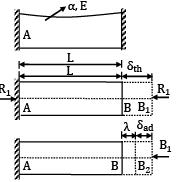BB1 = δth = α(∆t)L

B1 B2 = Expansion restricted by supports

λ = BB2 = yielding of support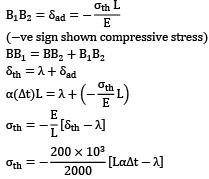= −100[(2000 x 10 x 10−6 x 40) − 0.2]

= −60 MPa = 60 MPa compressive

QUESTION: 18

Two steel rods of 20 mm diameter are joined end to end by means of a turn buckle as shown in figure, other end of each rod is rigidly fixed. Initially, there is no tension in each rod. Effective length of each rod is 5 m. Threads on each rod has a pitch of 2.4 mm. Calculate the increase in tension when the turn buckle is tightened by one third of revolution. E = 208 GPa. If the temperature of the rods is increased, then at what temperature, tension in each rod is reduced to half of its magnitude. αs = 11 x 10−6/℃.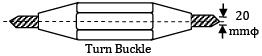Solution: A turn buckle is a type of nut having internal threads on two ends but in opposite directions. Ends of tie rods are inserted from both the sides as shown. By rotating the turn buckle, both the tie rods are tightened.

Length of each rod = 5 m Pitch of thread = 2.4 mm

Axial movement of rods on both the sides = 2.4/ 3 = 0.8 mm

Strain in each rod = 0.8 / 5000 = 0.16 x 10−3

E = 208000 N/mm2

σ, stress in each rod

= 0.16 x 10−3 x 208 x 1000 = 33.28 N/mm2

Area of cross–section of each rod,

A =π / 4 x 202 = 100 π mm2

Tension in each rod, T = 100π x 33.28

= 10455 N

= 10.455 kN

Tension in rod is to be reduced to half by increasing the temperature of the bar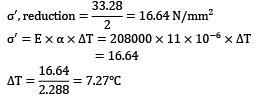QUESTION: 19

A rigid bar AOB, figure, pinned at O is attached to two vertical bars of steel and brass as shown. Initially the rigid bar is horizontal and vertical rods are stress free. If the temperature of the brass bar only increased by 50℃. Then stress developed in the steel bar is __________MPa.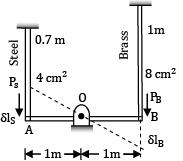Neglect weight of the bars.

LB = 1, LS = 0.7 m, AS = 400 mm2,

AB = 800 mm2, αb = 22.1 x 10-6/℃,

ES = 2EB = 200 GPa.

Solution: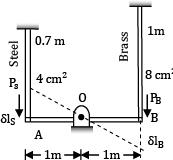Free expansion of brass bar is partially prevented, because it is connected to a steel bar through a rigid bar.

Say σBT = compressive stress in brass bar due to length change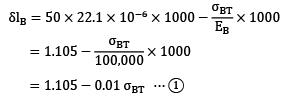Decrease in the length of steel bar,

?ls = ?lB

As AO = OB, (See the deformation triangles)

Say ?ST = compressive stress developed in steel bar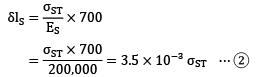Compressive force in brass bar = PB

Compressive force in steel bar = PS

PB x 1 = PS x 1

Or

PB = PS

σBT x 800 = σST x 400 ⋯ ③

or σBT = 0.5 σST ⋯ ④

Now δlS = δlB

1.105 − 0.01 σBT = 3.5 x 10−3 σST

But σBT = 0.5 σST

Therefore 1.105 − 0.01 / 2x σST

= 3.5 x 10−3 σST 1.105

= 8.5 x 10−3 σST

Stress σST = ?/?? (compressive)

σBT = 65 N/mm2 (compressive)

QUESTION: 20

A rigid plate ABCD, 1 m2 is supported over four legs of equal lengths of same crosssection and same material. A vertical load 1200 N acts at point E such that

AM = LE = 0.5 m

AL = ME = 0.4 m

The reactions RA , RB , RC and RD are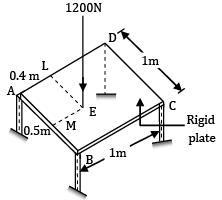Solution: For equilibrium RA + RB + RC + RD = 1200 N ⋯ ①

ABCD is a rigid plate, under load, its plane is changed, it is not deformed. Say under load legs deform by δA , δB , δC , δD respectively.

Diagonal AC and diagonal BD remain straight. Centre of the plate lies on both diagonals AC and BD.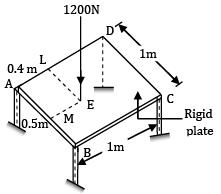δO = deflection at centre of plates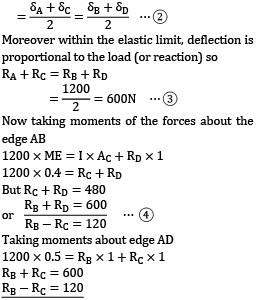Moreover within the elastic limit, deflection is proportional to the load (or reaction) so

RA + RC = RB + RD

= 1200 / 2

= 600N ⋯ ③

Now taking moments of the forces about the edge AB

1200 x ME = I x AC + RD x 1

1200 x 0.4 = RC + RD

But RC + RD = 480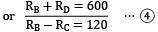1200 x 0.5 = RB x 1 + RC x 1

RB + RC = 600

RB − RC = 120

RB = 360N

RC = 240N

RA = 600 − RC

RA = 600 − 240 = 360N

RB + RD = 600

RD = 600 − RB

= 600 − 360 = 240N

Finally the reactions are RA = 360N,

RB = 360N, RC = 240N, RD = 240N